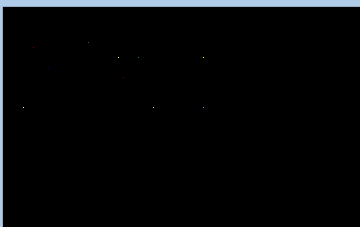# putpixel() function in C

• Difficulty Level : Medium
• Last Updated : 23 Jan, 2018

The header file graphics.h contains putpixel() function which plots a pixel at location (x, y) of specified color.
Syntax :

```void putpixel(int x, int y, int color);

where,
(x, y) is the location at which pixel
is to be put , and color specifies
the color of the pixel.
```

Explanation : A RED color pixel at (50, 40) can be drawn by using putpixel(50, 40, RED). putpixel() function can be used to draw circles, lines and ellipses using various algorithms.

Take a step-up from those "Hello World" programs. Learn to implement data structures like Heap, Stacks, Linked List and many more! Check out our Data Structures in C course to start learning today.

Below is the implementation of putpixel() function.

 `// C Implementation for putpixel()``#include ``#include `` ` `// driver code``int` `main()``{``    ``// gm is Graphics mode which is``    ``// a computer display mode that``    ``// generates image using pixels.``    ``// DETECT is a macro defined in``    ``// "graphics.h" header file``    ``int` `gd = DETECT, gm, color;`` ` `    ``// initgraph initializes the``    ``// graphics system by loading a``    ``// graphics driver from disk``    ``initgraph(&gd, &gm, ``""``);`` ` `    ``// putpixel function``    ``putpixel(85, 35, GREEN);``    ``putpixel(30, 40, RED);``    ``putpixel(115, 50, YELLOW);``    ``putpixel(135, 50, CYAN);``    ``putpixel(45, 60, BLUE);``    ``putpixel(20, 100, WHITE);``    ``putpixel(200, 100, LIGHTBLUE);``    ``putpixel(150, 100, LIGHTGREEN);``    ``putpixel(200, 50, YELLOW);``    ``putpixel(120, 70, RED);`` ` `    ``getch();`` ` `    ``// closegraph function closes the``    ``// graphics mode and deallocates``    ``// all memory allocated by``    ``// graphics system .``    ``closegraph();`` ` `    ``return` `0;``}`

Output :

``````
My Personal Notes arrow_drop_up